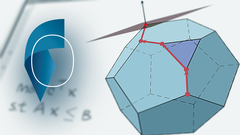## Linear and Discrete Optimization

 所在平台: Coursera 课程类别: 计算机科学Explore 1600+ online courses from top universities. Join Coursera today to learn data science, programming, business strategy, and more.#### 课程详情

This course serves as an introduction to linear and discrete optimization from the viewpoint of a mathematician or computer scientist.  Besides learning how linear and discrete optimization can be applied, we focus on understanding methods that solve linear programs and discrete optimization problems in a mathematically rigorous way.

• Does a particular method work correctly?
• Does it terminate and, if yes, in what time?
• Can we prove that a solution is optimal?
The course starts by discussing what a linear program is and how linear programming can be applied. Then, we will treat the simplex method and the theory of duality. We will then discuss some combinatorial optimization problems like maximum weight bipartite matching and maximum flows.

The course constitutes about half of the material on linear and discrete optimization that is taught for mathematics and computer science undergraduates at EPFL and will feature video lectures, quizzes, programming assignments, and a final exam.

#### 课程大纲

• Linear programming, modeling, equivalence of standard forms
• Basic solutions, primal and dual feasible basic solutions, pivoting and the simplex method
• Termination and complexity of the simplex method
• Integer programming, bipartite matching and flows
• Models of computation, bit-complexity

#### 课程评论(1条)

 0 wzyer 2013-05-17 09:15 0 票支持; 0 票反对 偏数学的课程。不过貌似优化本来也就是个数学问题。编程不难，难的是理解里边的数学思想。个人觉得还是很赞的一门课，我现在也没有完全吃透。期待会有什么凸优化之类的后续课程。